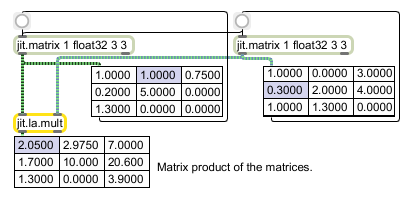# jit.la.mult

True matrix multiplication

## Description

The jit.la.mult object calculates the matrix product of two input matrices (as opposed to multiplication performed by the jit.op object, which is pointwise multiplication). The input matrices must have typefloat32 or float64, and may have planecount 1 or 2. If the input matrix has a planecount of 2, it is assumed that the data is from the set of complex numbers.

## Matrix Operator

matrix inputs:2, matrix outputs:1
 Name IOProc Planelink Typelink Dimlink Plane Dim Type in2 adapt 1 1 0 1 1 float32 float64 out n/a 1 1 0 1 1 float32 float64

## Examples